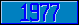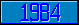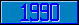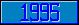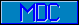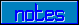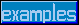## Indirection operator (@)

Introduced in the 1977 ANSI M[UMPS] language standard.

Three types of indirection:

Name indirection

Set X="ABC"
IF 123+@X=456

Argument indirection

Set SPACE="!!!",PAGE="#"
Write @\$Select(ENOUGH:SPACE,1:PAGE)

Pattern indirection

Set CODE="3U"_\$Select(SPECIAL:"2N",1:"")_"5L"
If X?@CODE

>Set string="123-44-5678"
>Write string?3N1"-"2N1"-"4N
1
>>Set pattern="3N1""-""2N1""-""4N"
>Write pattern
3N1"-"2N1"-"4N
>Write string?@pattern
1
>

Addition in 1984 ANSI M[UMPS] language standard.

Fourth type of indirection, subscripted reference indirection:

Set ARRAY="PRICES"
Set PRICE=(100+SALESTAX/100)*@ARRAY@(1,2,3)

Set ARRAY="^CUSTOMER(123,45)"
Set TOTAL=TOTAL+@ARRAY@(2,3,4)

Approved for addition in a future M[UMPS] Language standard.

Fifth type of indirection, "generic" indirection.

This new type of indirection involves a "catch-all" recovery after all other types of indirection have been attempted by a M[UMPS] language processor. When code is encountered that uses indirection, and none of the above forms of indirection leads to a valid interpretation of the code, the indirection operator and the expression on which it operates are to be replaced by the value of the expression in question, and then the line of code is to be re-evaluated. This may lead to some surprising possibilities:

Set X1="Y"
Set X2="Z="
Set X3="SecretAccnt=1E9,N"
Set X4="N=1 HALT"
Set Y="Example"
Set Y1="Example1"
Set Z1="Program"
Set Z2="TAG^Program"
Set Z3="(1,2,3)"
Set Z4=",2,3,4)"

With these values:

Set @X1="HELLO"
will be executed as: Set Y="HELLO"

Set @X2"HELLO"
will be executed as: Set Z="HELLO"

Set @X3="HELLO"
will be executed as: Set SecretAccnt=1E9,N="HELLO"

Set @(X1)1="New Value"
will be executed as: Set Y1="New Value"

Do ^@(Z1)(1,2,3)
will be executed as: Do ^Program(1,2,3)

Do @(Z2)(1,2,3)
will be executed as: Do TAG^Program(1,2,3)

Do @(Z2)@Z3
will be executed as: Do TAG^Program(1,2,3)

Do @(Z2)("BLUE"@Z4
will be executed as: Do TAG^Program("BLUE",2,3,4)